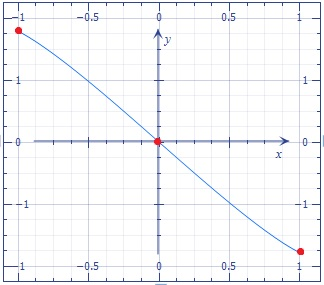# Find: Draw y=F(x)=\int _1^x (-1-\sqrt{1-t^2})dt with correct concavity on a scaled set of axes...

## Question:

Draw {eq}y=F(x)=\int _1^x (-1-\sqrt{1-t^2})dt {/eq} with correct concavity on a scaled set of axes (Include 3 labeled points)

## Concavity and Inflection point:

We can find the concavity and the inflection points of a function with the second derivative test, when we equal zero to the second derivative we find inflection points, at these points the concavity changes.

We have,

{eq}y=F(x)=\int _1^x (-1-\sqrt{1-t^2})dt \\ {/eq}

Leibniz formula for integrals:

{eq}f'(x)= \displaystyle \int_{g(x)}^{h(x)} f(t) \, dt = f(h(x))* \frac{dh}{dx} - f(g(x))* \frac{dg}{dx} \\ {/eq}

Applying The Leibniz formula:

{eq}f'(x)=-1-\sqrt{ 1-x^{2} } *1 -0 \\ f'(x)=-1-\sqrt{ 1-x^{2} } \\ {/eq}

Differentiating the function

{eq}f'(x)=-1-\sqrt{ 1-x^{2} } \\ f''(x)={\frac {x}{\sqrt {-{x}^{2}+1}}} \\ {/eq}

{eq}f''(x)=0 {/eq} when {eq}x=0 {/eq}

{eq}\begin{array}{r|D{.}{,}{5}} Interval & {-1<x<0} & {0<x<1} \\ \hline Test \space{} value & \ x=-0.5 & \ x=0.5 \\ Sign \space{} of \ f'' (x) & \ f'' (-0.5)<0 & \ f'' (-0.5)>0 \\ Conclusion & concave \space down & concave \space up \\ \end{array} \\ {/eq}

The inflection point at: {eq}x=0 {/eq}

We know that: {eq}y=F(x)=\int _1^x (-1-\sqrt{1-t^2})dt \\ \Rightarrow -x- \frac{1}{2}\,x\sqrt {- \left( x-1 \right) \left( x+1 \right) }-\frac{1}{2}\,\arcsin \left( x \right) \\ {/eq}

Draw the function: